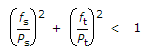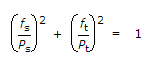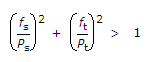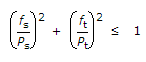# Civil Engineering - Steel Structure Design

1.
In a grillage footing, the maximum shear force occurs at the
edge of grillage beam
edge of base plate
centre of grillage beam
centre of base plate.
Explanation:
No answer description is available. Let's discuss.

2.
If d is the diameter of column, D is the side of square base, W is total axial load and p is the allowable bending stress, the thickness (t) of the base plate, is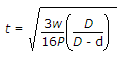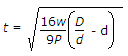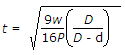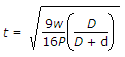Explanation:
No answer description is available. Let's discuss.

3.
Modified moment of inertia of sections with a single web, is equal to moment of inertia of the section about Y-Y axis at the point of maximum bending moment and is multiplied by the ratio of
area of compression flange at the minimum bending moment to the corresponding area at the point of maximum bending moment
area of tension flange at the minimum bending moment of the corresponding area at the point of maximum bending moment
total area of flanges at the maximum bending moment to the corresponding area at the point of maximum bending moment
none of these.
Explanation:
No answer description is available. Let's discuss.

4.
Rivets subjected to shear and an externally applied tensile force, should be so proportioned that